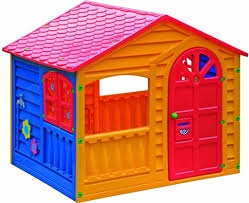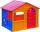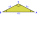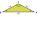# The gable

The gable of the house has the shape of an isosceles triangle, which has a base length of 14 meters and arms with a length of 8 meters. What is the tall gable of the house?

h =  3.873 m

### Step-by-step explanation:Did you find an error or inaccuracy? Feel free to write us. Thank you!Tips to related online calculators
Pythagorean theorem is the base for the right triangle calculator.

#### You need to know the following knowledge to solve this word math problem:

We encourage you to watch this tutorial video on this math problem:

## Related math problems and questions:

• Face of the houseHow tall is the gable of a house in the shape of an isosceles triangle with a base length of 8 meters and an arm 5 meters long?
• Right isosceles triangleWhat can be the area of a right isosceles triangle with a side length of 8 cm?
• Isosceles trapezoidWhat is the height of an isosceles trapezoid, the base of which has a length of 11 cm and 8 cm and whose legs measure 2.5 cm?
• CalculateCalculate the height to the base of the isosceles triangle ABC if the length of the base is c = 24cm and the arms have a length b = 13cm.
• Isosceles triangleCalculate the area of an isosceles triangle, the base of which measures 16 cm and the arms 10 cm.
• Base of an isosceles triangleCalculate the size of the base of an isosceles triangle, the height is 5 cm and the length of the arm is 6.5 cm. What is the perimeter of this triangle?
• Isosceles right triangleArea of an isosceles right triangle is 18 dm2. Calculate the length of its base.
• Isosceles triangleCalculate area and perimeter of an isosceles triangle ABC with base AB if a = 6 cm, c = 7 cm.
• Right isoscelesCalculate area of the isosceles right triangle which perimeter is 26 cm.
• Roof angleThe roof of the house has the shape of an isosceles triangle with arms 4 m long and the size of the base 6 m. How big an angle alpha does its roof make?
• CalculateCalculate the height of an isosceles triangle with base 37.8 mm long and an arm 23.1 mm long.
• SquareCalculate the area of the square shape of the isosceles triangle with the arms 50m and the base 60m. How many tiles are used to pave the square if the area of one tile is 25 dm2?
• Isosceles + prismCalculate the volume of the perpendicular prism if its height is 17.5 cm and the base is an isosceles triangle with a base length of 5.8 cm and an arm length of 3.7 cm
• The regularThe regular triangular prism has a base in the shape of an isosceles triangle with a base of 86 mm and 6.4 cm arms, the height of the prism is 24 cm. Calculate its volume.
• Railway embankmentThe railway embankment section is an isosceles trapezoid, the sizes of the bases of which are in the ratio 5: 3. The arms have a length of 5 m, and the height of the embankment is 4.8 m. Calculates the size of the embankment section area.
• Isosceles trapezoidFind the area of an isosceles trapezoid, if the bases are 12 cm and 20 cm, the length of the arm is 16 cm
• The right triangleThe right triangle ABC has a leg a = 36 cm and an area S = 540 cm2. Calculate the length of the leg b and the median t2 to side b.